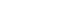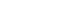# GMAT Math Equations

Here’s a brief rundown of important GMAT math equations to know.  This outline will follow the organization of the “Math Review” in the GMAT OG.

## Arithmetic

It’s not really an equation, but you should know how to add & subtract & multiply & divide fractions.   Percents are all over the GMAT, and you should understand converting among decimals & fractions & percents, as well as the crucial topic of percent changes.  Among the big arithmetic equations are:

= the “remainder” equation (a.k.a. “rebuilding the dividend”)

= the doubling and halving method

= the average formula (esp. for finding the sum)

Notice, I do not list standard deviation as an equation you need to know — you need to understand the properties of standard deviation, but not the exact formula.

## Algebra

It’s not really an equation, but you should understand the procedures for solving for x, or solving for two variables with two equations.  You should understand the rules for inequalities.  Ironically, I will not recommend perhaps the most famous high school algebra equation, the Quadratic Formula — it’s much more important on the GMAT to know how to solve quadratics by factoring.  Algebra equations important on the GMAT include:

= absolute values and negatives produce befuddling equations

## Geometry

The beautiful realm of geometry entails several important formulas, starting with the great granddaddy of them all:

others include:

= area of a triangle (A = 0.5bh)

= area of a rectangle (A = bh)

== Archimedes’ formula:= Volume of a box = (height)*(width)*(depth)

= slope in the x-y plane

Oddly, I am going to strongly recommend NOT to memorize a formula for finding distance in the x-y plane — I explain what to do instead at that link.  🙂

## Word Problems

= rate, distance, and time

= (profit) = (revenue) – (cost)

Problems of interest and discount just involve the same percent equations discussed above.

How to study for GMAT math

Mathematical thinking on the GMAT

If you have questions or feedback about the GMAT math equations you’ll need to know, please let us know in the comments section below.

## Author

•Mike served as a GMAT Expert at Magoosh, helping create hundreds of lesson videos and practice questions to help guide GMAT students to success. He was also featured as "member of the month" for over two years at GMAT Club. Mike holds an A.B. in Physics (graduating magna cum laude) and an M.T.S. in Religions of the World, both from Harvard. Beyond standardized testing, Mike has over 20 years of both private and public high school teaching experience specializing in math and physics. In his free time, Mike likes smashing foosballs into orbit, and despite having no obvious cranial deficiency, he insists on rooting for the NY Mets. Learn more about the GMAT through Mike's Youtube video explanations and resources like What is a Good GMAT Score? and the GMAT Diagnostic Test.